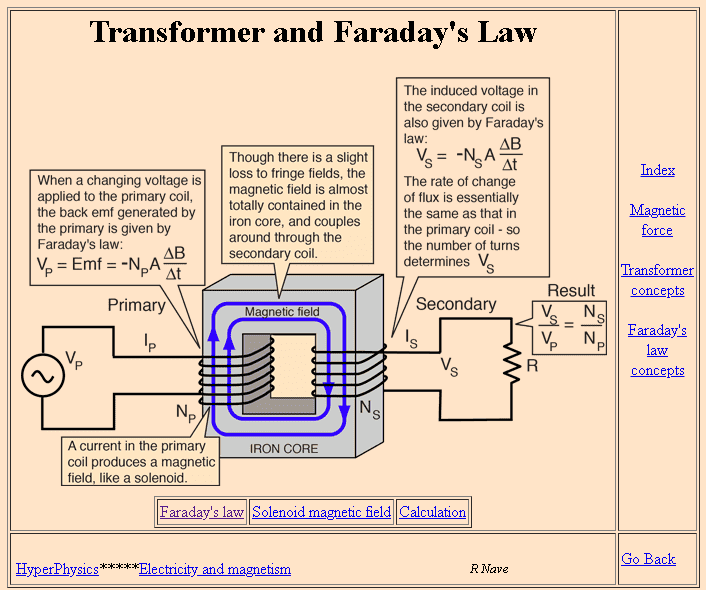# Transformer: single primary coil and multiple secondary coils

If I have a coil with 10 turns and a primary voltage $$V_p$$ and primary current $$I_p$$ and a single secondary coil with 10 turns, I understand the power in the secondary will be $$P=V_pI_P$$ and hence voltage and current in the secondary coil will be the same.

If I now bring a 3rd coil with 10 turns into the mix, what will be the voltage in and current in the secondary and tertiary coil? My intuition says that the voltage will be the same and equal to $$V_p$$ in the secondary and tertiary coil but the current will be halved $$I_p/2$$ in both in order to conserve energy.

Is this correct? It makes me wonder how the third coil would "know" about the existence of the second coil and realise how to change its current in response to it.

Rive
The current on a coil (of the transformer) is not determined by the coil alone. The turn ratios will tell you the voltages, and then you have to calculate the currents based on the load.
With that you can sum up the power on the secondary, then determine the current on the primary (if the power is still within the specification of the transformer, and the currents are within the specification of the relevant coil).
If you have values out of spec that is a different story.

•Fisherman199, Merlin3189 and anorlunda
berkeman
Mentor
If I now bring a 3rd coil with 10 turns into the mix, what will be the voltage in and current in the secondary and tertiary coil? My intuition says that the voltage will be the same
You need to include the source impedance of the signal source driving the primary winding, and the load impedance on each of the output windings, in addition to the turns ratios.

The load impedances transform back (via a version of the turns ratio -- can you say what that ratio is?) to the primary side, and load the signal source accordingly. To calculate the secondary output voltages and currents, you need to solve for the whole system working together.

And then when you are ready for the next layer of the model, you will include the effects of the leakage inductance (Lk) of each winding, versus the magnetizing inductances (Lm) that couple the B-field between the coils...

jim hardy
Gold Member
Dearly Missed
It makes me wonder how the third coil would "know" about the existence of the second coil and realise how to change its current in response to it.

Hmmm we are speaking of a traditional iron core power transformer here, not an air core signal transformer ?
And sinewave excitation ?

The third coil is oblivious to all other coils.
In transformers you sum the currents, see belowThe primary coil establishes magnetic flux in the core.
The magnitude of that flux you can measure as volts per turn
and so long as primary voltage is constant so will be flux
That flux passes also through the secondary winding where it induces voltage
if any current can flow in the secondary,
the current there makes a second magnetic flux opposing that from the primary
reducing total flux
so more current will flow into primary to keep total flux constant.

Adding a second secondary doesn't change a thing .
Current in that secondary will also result in more primary current.

In an unloaded power transformers the product of amps X turns will be constant, and a very small number (in fact for an ideal transformer it'll be zero)
and when you load it the SUM [(primary amp-turns) - (Σsecondary amp turns)] will be the same small number (or zero if an ideal transformer)..

That approach will lead your brain to the right equations. Practice it.

Any help ?

old jim

#### Attachments

•dlgoff